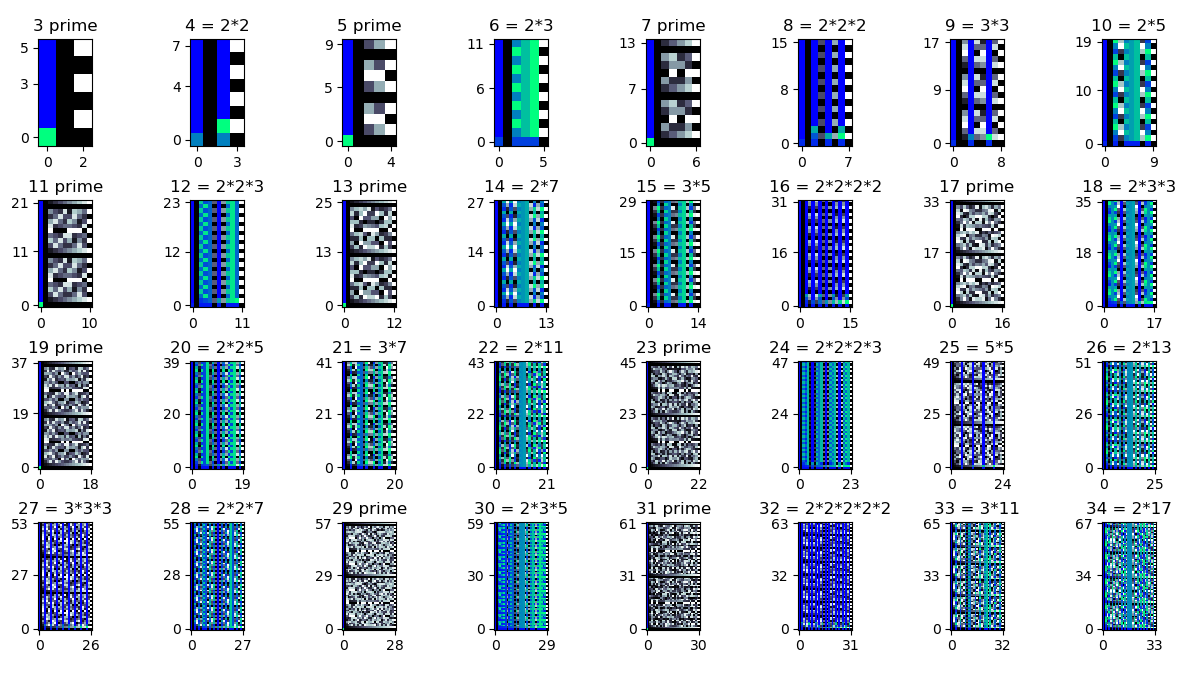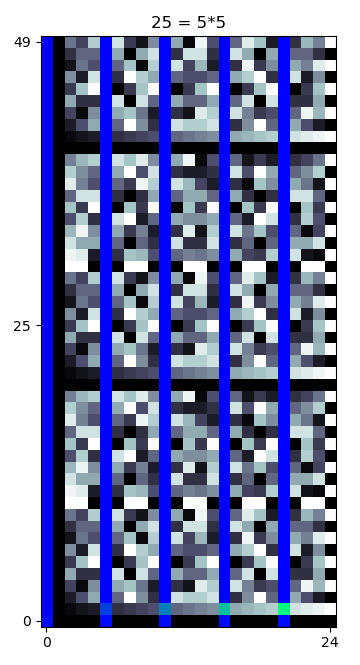I recently encountered the following story on Stack Exchange:

In 1969, D. J. Lewis wrote a paper about Diophantine equations, in which he wrote that the equation $$x^3 + 5 = 117 y^3$$ is known to have at most 18 solutions, but the exact number is not known. Two other mathematicians studied the equation and, in 1971, they published a short but difficult proof that there are no solutions. Finally, in 1973, another mathematician found an astonishingly simple proof of the same fact! The proof is:

The quantity $$x^3 + 5$$ is never a multiple of 9, but the quantity $$117 y^3$$ is always a multiple of 9, so there are no solutions.

I decided to try to verify the short proof at the end of this amusing anecdote. It is easy to show that $$117 y^3$$ is always a multiple of 9, because 117 is a multiple of 9. It is somewhat less obvious that $$x^3 + 5$$ is never a multiple of 9. This statement is equivalent to $$x^3 \not\equiv 4\ (\text{mod}\ 9)$$. I couldn’t come up with an easier way to prove this than just checking all 9 congruence classes individually.

We have:

• $$0^3 \equiv 0\ (\text{mod}\ 9)$$
• $$1^3 \equiv 1\ (\text{mod}\ 9)$$
• $$2^3 \equiv 8\ (\text{mod}\ 9)$$
• $$3^3 \equiv 0\ (\text{mod}\ 9)$$
• $$4^3 \equiv 1\ (\text{mod}\ 9)$$
• $$5^3 \equiv 8\ (\text{mod}\ 9)$$
• $$6^3 \equiv 0\ (\text{mod}\ 9)$$
• $$7^3 \equiv 1\ (\text{mod}\ 9)$$
• $$8^3 \equiv 8\ (\text{mod}\ 9)$$

When I wrote this out, I noticed an interesting pattern. If we evaluate $$a^3\ \text{mod}\ 9$$ for $$a = 0,1,2,\dots$$, we get a repeating cycle of $$[0, 1, 8]$$. This made me wonder whether this was a coincidence or if there was something more here. There are of course many well-known theorems about congruences of integer powers, such as Fermat’s little theorem, but I wasn’t familiar with this cyclic behavior.

To look for more general patterns, I wrote a Python script that uses matplotlib to graph the value of $$a^b\ \text{mod}\ m$$ for different values of $$a$$, $$b$$, and $$m$$. Each subplot graphs the values of the function for a single $$m$$ value. The x-axis is (congruence classes of) $$a$$, while the y-axis is $$b$$. Columns where $$a$$ is coprime to $$m$$ are shown in black and white, while columns where $$a$$ shares a factor with $$m$$ are shown in color. The 3-cycle descibed above is visible as a repeating pattern of 3 colors in the row fourth from the bottom in the plot for $$m = 9$$.Plots of modpow function for $$m = 3$$ through $$34$$. (full size version)

I noticed that there was also a repeating cycle of 5 elements with $$b = 5, m = 25$$, similar to the previous repeating cycle with $$b = 3, m = 9$$.Zoom on plot of modpow function for $$m = 25$$.

As it turns out, this pattern generalizes beyond $$9 = 3^2$$ and $$25 = 5^2$$. I was able to prove the following:

Theorem: $$a^b\ \text{mod}\ b^2$$ is $$b$$-periodic in $$a$$. In other words, $$(a+b)^b \equiv a^b\ (\text{mod}\ b^2)$$.

Proof: By the binomial theorem, $$(a + b)^b$$ expands into a sum of monomials $\sum_{k = 0}^{b} \binom{b}{k}\,a^{b - k}\,b^k.$ Any term with $$k \ge 2$$ will be divisible by $$b^2$$ and hence will not contribute to the final sum. So the final result will be $\binom{b}{0}\,a^{b - 0}\,b^0 + \binom{b}{1}\,a^{b - 1}\,b^1 = a^b + b\,a^{b - 1}\,b.$ The last term is also divisible by $$b^2$$ and hence will not contribute to the final sum. So the final result is just $$a^b$$.

Yay, math!